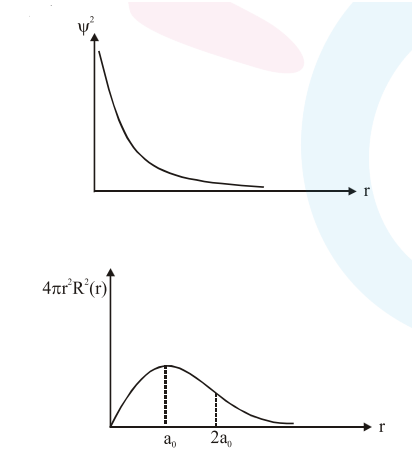# Which one of the following about an electron occupying

Question:

Which one of the following about an electron occupying the $1 \mathrm{~s}$ orbital in a hydrogen atom is incorrect ? (The Bohr radius is represented by $\mathrm{a}_{0}$ )

1. The electron can be found at a distance $2 \mathrm{a}_{0}$ from the nucleus

2. The probability density of finding the electron is maximum at the nucleus.

3. The magnitude of potential energy is double that of its kinetic energy on an average.

4. The total energy of the electron is maximum when it is at a distance $a_{0}$ from the nucleus.

Correct Option: , 4

Solution: